Maths-
General
Easy

Question

# The area bounded by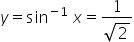and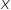-axis is

##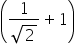sq unit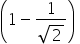sq unit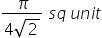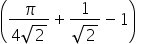sq unitHint:

## The correct answer is:sq unit

###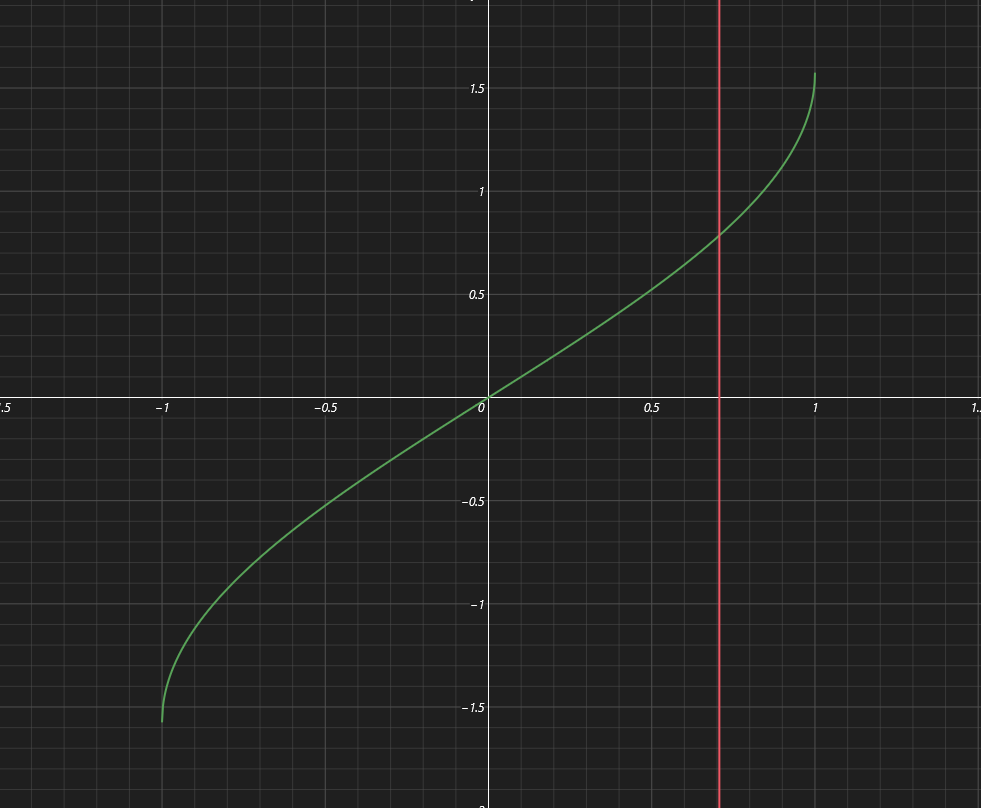In This figure the green curve is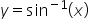and the red line is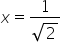We have to find the area bounded inside the point of intersection of these two curves and above the x-axisThe point of intersection is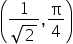The area will be equal to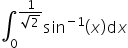We know that for integration of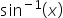we will use ILATE rule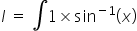let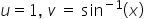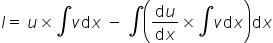By substituting the values of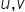in the above equation we get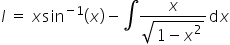Let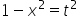=> By differentiating both sides we get,=>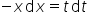=>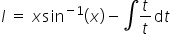=>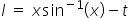=>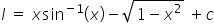where c is the constant of Integration.By putting the limits we get=>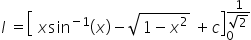=>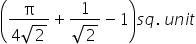#### With Turito Foundation.#### Get an Expert Advice From Turito.